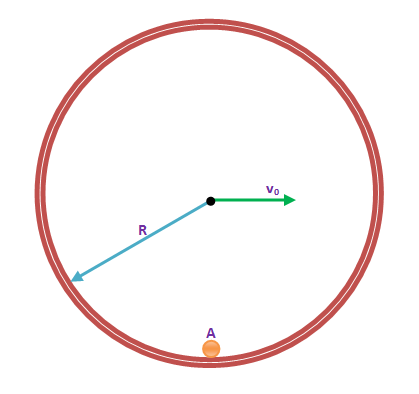A small body A is fixed to the inner side of a thin rigid hoop of radius R and mass equal to that of the body A. The hoop rolls without slipping over a horizontal plane. At the moments when the body A gets into the lower position, the centre of the hoop moves with velocity $V_{o}$ . The maximum value of $V_{o}$ at which the hoop moves without bouncing is $\sqrt{xgR}$. What is the value of $'x'$?